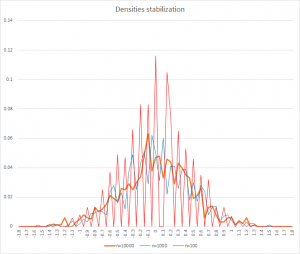4
Sep 16

## The pearls of AP Statistics 25### Central Limit Theorem versus Law of Large Numbers

They say: The Central Limit Theorem (CLT). Describes the Expected Shape of the Sampling Distribution for Sample Mean$\bar{X}$. For a random sample of size$n$ from a population having mean μ and standard deviation σ, then as the sample size$n$ increases, the sampling distribution of the sample mean$\bar{X}$ approaches an approximately normal distribution. (Agresti and Franklin, p.321)

I say: There are at least three problems with this statement.

Problem 1. With any notion or statement, I would like to know its purpose in the first place. The primary purpose of the law of large numbers is to estimate population parameters. The Central Limit Theorem may be a nice theoretical result, but why do I need it? The motivation is similar to the one we use for introducing the z score. There is a myriad of distributions. Only some standard distributions have been tabulated. Suppose we have a sequence of variables$X_n$, none of which have been tabulated. Suppose also that, as$n$ increases, those variables become close to a normal variable in the sense that the cumulative probabilities (areas under their respective densities) become close:

(1)$P(X_n\le a)\rightarrow P(normal\le a)$ for all$a$.

Then we can use tables developed for normal variables to approximate$P(X_n\le a)$. This justifies using (1) as the definition of a new convergence type called convergence in distribution.

Problem 2. Having introduced convergence (1), we need to understand what it means in terms of densities (distributions). As illustrated in Excel, the law of large numbers means convergence to a spike. In particular, the sample mean converges to a mass concentrated at μ (densities contract to one point). Referring to the sample mean in the context of CLT is misleading, because the CLT is about densities stabilization.Figure 1. Law of large numbers with n=100, 1000, 10000

Figure 1 appeared in my posts before, I just added n=10,000, to show that densities do not stabilize.Figure 2. Central limit theorem with n=100, 1000, 10000

In Figure 2, for clarity I use line plots instead of histograms. The density for n=100 is very rugged. The blue line (for n=1000) is more rugged than the orange (for n=10,000). Convergence to a normal shape is visible, although slow.

Main problem. It is not the sample means that converge to a normal distribution. It is their z scores$z=\frac{\bar{X}-E\bar{X}}{\sigma(\bar{X})}$

that do. Specifically,$P(\frac{\bar{X}-E\bar{X}}{\sigma(\bar{X})}\le a)\rightarrow P(z\le a)$ for all$a$

where$z$ is a standard normal variable.

In my simulations I used sample means for Figure 1 and z scores of sample means for Figure 2. In particular, z scores always have means equal to zero, and that can be seen in Figure 2. In your class, you can use the Excel file. As usual, you have to enable macros.